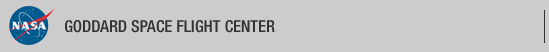+ Visit NASA.gov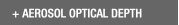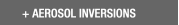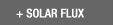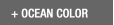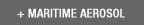Web Site Feature AERONET Data Synergy Tool - Access Earth Science data sets for AERONET sites+Home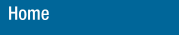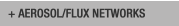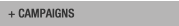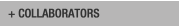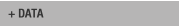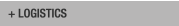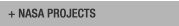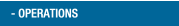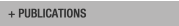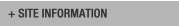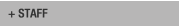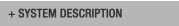AERONET DATA ACCESS

AERONET Site Lists (V3)

+ All Lists# Report of DCP transmissions

 Status Legend

AERONET No 930 - 112 msg rcvd (45430 b) site : Socheongcho

# Aeronet No 930 (Socheongcho)

11:12:2019,05:31:00 (UTC) (DCP total: 647) Cimel : 930 time corr. = 65 sec all(3) alr(3) all(4) alr(4) nsu pp1(1)
11:12:2019,06:01:00 (UTC) (DCP total: 647) Cimel : 930 time corr. = 65 sec pp1(2) pp1(3) pp1(4) blk staP nsu prs(1) prs(2)
11:12:2019,06:31:00 (UTC) (DCP total: 647) Cimel : 930 time corr. = 65 sec prs(3) prs(4) prs(5) prs(6) prs(8) prs(9) staD nsu staD nsu staP nsu
11:12:2019,07:01:00 (UTC) (DCP total: 647) Cimel : 930 time corr. = 65 sec prs(1) prs(2) prs(3) prs(4) prs(5) prs(6) prs(8) prs(9) staD nsu staF
11:12:2019,07:31:00 (UTC) (DCP total: 647) Cimel : 930 time corr. = 65 sec nsu all(1) alr(1) all(2) alr(2) all(3) alr(3)
11:12:2019,08:01:00 (UTC) (DCP total: 647) Cimel : 930 time corr. = 65 sec all(4) alr(4) nsu pp1(1) pp1(2) pp1(3)
11:12:2019,08:31:00 (UTC) (DCP total: 647) Cimel : 930 time corr. = 65 sec pp1(4) blk staP nsu prs(1) prs(2) prs(3) prs(4) prs(5) prs(6) prs(8)
11:12:2019,09:01:00 (UTC) (DCP total: 647) Cimel : 930 time corr. = 65 sec prs(9) staD nsu staD nsu staP nsu prs(1) prs(2) prs(3) prs(4) prs(5)
11:12:2019,09:31:00 (UTC) (DCP total: 647) Cimel : 930 time corr. = 65 sec prs(6) prs(8) prs(9) staD nsu staF nsu all(1) alr(1)
11:12:2019,10:01:00 (UTC) (DCP total: 647) Cimel : 930 time corr. = 65 sec all(2) alr(2) all(3) alr(3) all(4) alr(4) nsu
11:12:2019,10:31:00 (UTC) (DCP total: 647) Cimel : 930 time corr. = 65 sec pp1(1) pp1(2) pp1(3) pp1(4) blk staP nsu
11:12:2019,11:01:00 (UTC) (DCP total: 647) Cimel : 930 time corr. = 65 sec prs(1) prs(2) prs(3) prs(4) prs(5) prs(6) prs(8) prs(9) staD nsu staD nsu
11:12:2019,11:31:00 (UTC) (DCP total: 647) Cimel : 930 time corr. = 65 sec staP nsu prs(1) prs(2) prs(3) prs(4) prs(5) prs(6) prs(8) prs(9) staD nsu
11:12:2019,12:01:00 (UTC) (DCP total: 647) Cimel : 930 time corr. = 65 sec staF nsu staP nsu prs(1) prs(2) prs(3) prs(4) prs(5) prs(6) prs(8)
11:12:2019,12:31:00 (UTC) (DCP total: 647) Cimel : 930 time corr. = 65 sec prs(9) staD nsu staD nsu staP nsu staD nsu staF nsu staP
11:12:2019,13:01:00 (UTC) (DCP total: 647) Cimel : 930 time corr. = 65 sec nsu staA nsu staA nsu staB nsu staA nsu staP nsu
11:12:2019,13:31:00 (UTC) (DCP total: 647) Cimel : 930 time corr. = 65 sec prs(1) prs(2) prs(3) prs(4) prs(5) prs(6) prs(8) prs(9) staA nsu staA nsu staA
11:12:2019,14:00:00 (UTC) (DCP total: 370) Cimel : 930 time corr. = 65 sec (DCP Volt = 12.10 Lat= 37.423 Long= 124.738(0.0 km from Socheongcho)) nsu staA nsu staA
11:12:2019,14:30:00 (UTC) (DCP total: 92) Cimel : 930 time corr. = 65 sec (DCP Volt = 12.00 Lat= 37.423 Long= 124.738(0.0 km from Socheongcho))
11:12:2019,15:00:00 (UTC) (DCP total: 92) Cimel : 930 time corr. = 65 sec (DCP Volt = 12.10 Lat= 37.423 Long= 124.738(0.0 km from Socheongcho))
11:12:2019,15:30:00 (UTC) (DCP total: 92) Cimel : 930 time corr. = 65 sec (DCP Volt = 12.10 Lat= 37.423 Long= 124.738(0.0 km from Socheongcho))
11:12:2019,16:00:00 (UTC) (DCP total: 92) Cimel : 930 time corr. = 65 sec (DCP Volt = 12.10 Lat= 37.423 Long= 124.738(0.0 km from Socheongcho))
11:12:2019,16:30:00 (UTC) (DCP total: 92) Cimel : 930 time corr. = 65 sec (DCP Volt = 12.10 Lat= 37.423 Long= 124.738(0.0 km from Socheongcho))
11:12:2019,17:00:00 (UTC) (DCP total: 92) Cimel : 930 time corr. = 65 sec (DCP Volt = 12.10 Lat= 37.423 Long= 124.738(0.0 km from Socheongcho))
11:12:2019,17:30:00 (UTC) (DCP total: 92) Cimel : 930 time corr. = 65 sec (DCP Volt = 12.10 Lat= 37.423 Long= 124.738(0.0 km from Socheongcho))
11:12:2019,18:00:00 (UTC) (DCP total: 92) Cimel : 930 time corr. = 65 sec (DCP Volt = 12.10 Lat= 37.423 Long= 124.738(0.0 km from Socheongcho))
11:12:2019,18:30:00 (UTC) (DCP total: 92) Cimel : 930 time corr. = 65 sec (DCP Volt = 12.10 Lat= 37.423 Long= 124.738(0.0 km from Socheongcho))
11:12:2019,19:00:00 (UTC) (DCP total: 92) Cimel : 930 time corr. = 65 sec (DCP Volt = 12.10 Lat= 37.423 Long= 124.738(0.0 km from Socheongcho))
11:12:2019,19:30:00 (UTC) (DCP total: 92) Cimel : 930 time corr. = 65 sec (DCP Volt = 12.10 Lat= 37.423 Long= 124.738(0.0 km from Socheongcho))
11:12:2019,20:00:00 (UTC) (DCP total: 92) Cimel : 930 time corr. = 65 sec (DCP Volt = 12.10 Lat= 37.423 Long= 124.738(0.0 km from Socheongcho))
11:12:2019,20:30:00 (UTC) (DCP total: 92) Cimel : 930 time corr. = 65 sec (DCP Volt = 12.10 Lat= 37.423 Long= 124.738(0.0 km from Socheongcho))
11:12:2019,21:00:00 (UTC) (DCP total: 92) Cimel : 930 time corr. = 65 sec (DCP Volt = 12.10 Lat= 37.423 Long= 124.738(0.0 km from Socheongcho))
11:12:2019,21:30:00 (UTC) (DCP total: 92) Cimel : 930 time corr. = 65 sec (DCP Volt = 12.10 Lat= 37.423 Long= 124.738(0.0 km from Socheongcho))
11:12:2019,22:00:00 (UTC) (DCP total: 92) Cimel : 930 time corr. = 65 sec (DCP Volt = 12.10 Lat= 37.423 Long= 124.738(0.0 km from Socheongcho))
11:12:2019,22:30:00 (UTC) (DCP total: 92) Cimel : 930 time corr. = 65 sec (DCP Volt = 12.10 Lat= 37.423 Long= 124.738(0.0 km from Socheongcho))
11:12:2019,23:00:00 (UTC) (DCP total: 92) Cimel : 930 time corr. = 65 sec (DCP Volt = 12.10 Lat= 37.423 Long= 124.738(0.0 km from Socheongcho))
11:12:2019,23:30:00 (UTC) (DCP total: 92) Cimel : 930 time corr. = 65 sec (DCP Volt = 12.10 Lat= 37.423 Long= 124.738(0.0 km from Socheongcho))
12:12:2019,00:00:00 (UTC) (DCP total: 512) Cimel : 930 time corr. = 65 sec (DCP Volt = 12.10 Lat= 37.423 Long= 124.738(0.0 km from Socheongcho)) nsu staA nsu staA nsu staA nsu staA
12:12:2019,00:31:00 (UTC) (DCP total: 647) Cimel : 930 time corr. = 65 sec nsu staA nsu staP nsu prs(1) prs(2) prs(3) prs(4) prs(5) prs(6) prs(8)
12:12:2019,01:01:00 (UTC) (DCP total: 647) Cimel : 930 time corr. = 65 sec prs(9) staA nsu staB nsu all(1) alr(1) all(2) alr(2)
12:12:2019,01:31:00 (UTC) (DCP total: 647) Cimel : 930 time corr. = 65 sec all(3) alr(3) all(4) alr(4) staA nsu staA nsu staF
12:12:2019,02:01:00 (UTC) (DCP total: 647) Cimel : 930 time corr. = 65 sec nsu all(1) alr(1) all(2) alr(2) all(3)
12:12:2019,02:31:00 (UTC) (DCP total: 647) Cimel : 930 time corr. = 65 sec alr(3) all(4) alr(4) nsu pp1(1) pp1(2)
12:12:2019,03:01:00 (UTC) (DCP total: 647) Cimel : 930 time corr. = 65 sec pp1(3) pp1(4) blk staP nsu prs(1) prs(2) prs(3) prs(4) prs(5) prs(6)
12:12:2019,03:31:00 (UTC) (DCP total: 647) Cimel : 930 time corr. = 65 sec prs(8) prs(9) staD nsu staD nsu staP nsu prs(1) prs(2) prs(3)
12:12:2019,04:01:00 (UTC) (DCP total: 647) Cimel : 930 time corr. = 65 sec prs(4) prs(5) prs(6) prs(8) prs(9) staD nsu staF nsu all(1)
12:12:2019,04:31:00 (UTC) (DCP total: 647) Cimel : 930 time corr. = 65 sec alr(1) all(2) alr(2) all(3) alr(3) all(4) alr(4)
12:12:2019,05:01:00 (UTC) (DCP total: 647) Cimel : 930 time corr. = 65 sec nsu pp1(1) pp1(2) pp1(3) pp1(4) blk staP
12:12:2019,05:31:00 (UTC) (DCP total: 647) Cimel : 930 time corr. = 65 sec nsu prs(1) prs(2) prs(3) prs(4) prs(5) prs(6) prs(8) prs(9) staD nsu staD
12:12:2019,06:01:00 (UTC) (DCP total: 647) Cimel : 930 time corr. = 65 sec nsu staP nsu prs(1) prs(2) prs(3) prs(4) prs(5) prs(6) prs(8) prs(9) staD
12:12:2019,06:31:00 (UTC) (DCP total: 647) Cimel : 930 time corr. = 65 sec nsu staF nsu staP nsu prs(1) prs(2) prs(3) prs(4) prs(5) prs(6)
12:12:2019,07:01:00 (UTC) (DCP total: 647) Cimel : 930 time corr. = 65 sec prs(8) prs(9) staD nsu staD nsu staP nsu prs(1) prs(2) prs(3) prs(4)
12:12:2019,07:31:00 (UTC) (DCP total: 647) Cimel : 930 time corr. = 65 sec prs(5) prs(6) prs(8) prs(9) staD nsu staF nsu all(1) alr(1)
12:12:2019,08:01:00 (UTC) (DCP total: 647) Cimel : 930 time corr. = 65 sec all(2) alr(2) all(3) alr(3) all(4) alr(4)
12:12:2019,08:31:00 (UTC) (DCP total: 647) Cimel : 930 time corr. = 65 sec nsu pp1(1) pp1(2) pp1(3) pp1(4) blk staP
12:12:2019,09:01:00 (UTC) (DCP total: 647) Cimel : 930 time corr. = 65 sec nsu prs(1) prs(2) prs(3) prs(4) prs(5) prs(6) prs(8) prs(9) staD nsu staD
12:12:2019,09:31:00 (UTC) (DCP total: 647) Cimel : 930 time corr. = 65 sec nsu staP nsu staD nsu staF nsu staP nsu prs(1) prs(2)
12:12:2019,10:01:00 (UTC) (DCP total: 647) Cimel : 930 time corr. = 65 sec prs(3) prs(4) prs(5) prs(6) prs(8) prs(9) staD nsu staD nsu staP nsu
12:12:2019,10:31:00 (UTC) (DCP total: 647) Cimel : 930 time corr. = 65 sec prs(1) prs(2) prs(3) prs(4) prs(5) prs(6) prs(8) prs(9) staD nsu staF nsu
12:12:2019,11:01:00 (UTC) (DCP total: 647) Cimel : 930 time corr. = 65 sec all(1) alr(1) all(2) alr(2) all(3) alr(3)
12:12:2019,11:31:00 (UTC) (DCP total: 647) Cimel : 930 time corr. = 65 sec all(4) alr(4) nsu pp1(1) pp1(2) pp1(3)
12:12:2019,12:01:00 (UTC) (DCP total: 1991) Cimel : 930 time corr. = 65 sec pp1(4) blk staP nsu prs(1) prs(2) prs(3) prs(4) prs(5) prs(6) prs(8)
12:12:2019,12:31:00 (UTC) (DCP total: 1991) Cimel : 930 time corr. = 65 sec prs(9) staD nsu staD nsu staP nsu prs(1) prs(2) prs(3) prs(4) prs(5)
12:12:2019,13:01:00 (UTC) (DCP total: 1991) Cimel : 930 time corr. = 65 sec prs(6) prs(8) prs(9) staD nsu staF nsu all(1) alr(1)
12:12:2019,13:31:00 (UTC) (DCP total: 1991) Cimel : 930 time corr. = 65 sec all(2) alr(2) all(3) alr(3) all(4) alr(4) nsu staP
12:12:2019,14:01:00 (UTC) (DCP total: 1991) Cimel : 930 time corr. = 65 sec nsu prs(1) prs(2) prs(3) prs(4) prs(5) prs(6) prs(8) prs(9) staA nsu staA
12:12:2019,14:31:00 (UTC) (DCP total: 1991) Cimel : 930 time corr. = 65 sec nsu staB nsu staA nsu staP nsu prs(1) prs(2) prs(3) prs(4)
12:12:2019,15:01:00 (UTC) (DCP total: 1991) Cimel : 930 time corr. = 65 sec prs(5) prs(6) prs(8) prs(9) staA nsu staA nsu staA nsu staA nsu
12:12:2019,15:30:00 (UTC) (DCP total: 626) Cimel : 930 time corr. = 65 sec (DCP Volt = 12.10 Lat= 37.423 Long= 124.738(0.0 km from Socheongcho)) staA
12:12:2019,16:00:00 (UTC) (DCP total: 326) Cimel : 930 time corr. = 65 sec (DCP Volt = 12.00 Lat= 37.423 Long= 124.738(0.0 km from Socheongcho))
12:12:2019,16:30:00 (UTC) (DCP total: 326) Cimel : 930 time corr. = 65 sec (DCP Volt = 12.00 Lat= 37.423 Long= 124.738(0.0 km from Socheongcho))
12:12:2019,17:00:00 (UTC) (DCP total: 326) Cimel : 930 time corr. = 65 sec (DCP Volt = 12.10 Lat= 37.423 Long= 124.738(0.0 km from Socheongcho))
12:12:2019,17:30:00 (UTC) (DCP total: 326) Cimel : 930 time corr. = 65 sec (DCP Volt = 12.10 Lat= 37.423 Long= 124.738(0.0 km from Socheongcho))
12:12:2019,18:00:00 (UTC) (DCP total: 326) Cimel : 930 time corr. = 65 sec (DCP Volt = 12.10 Lat= 37.423 Long= 124.738(0.0 km from Socheongcho))
12:12:2019,18:30:00 (UTC) (DCP total: 326) Cimel : 930 time corr. = 65 sec (DCP Volt = 12.10 Lat= 37.423 Long= 124.738(0.0 km from Socheongcho))
12:12:2019,19:00:00 (UTC) (DCP total: 326) Cimel : 930 time corr. = 65 sec (DCP Volt = 12.10 Lat= 37.423 Long= 124.738(0.0 km from Socheongcho))
12:12:2019,19:30:00 (UTC) (DCP total: 326) Cimel : 930 time corr. = 65 sec (DCP Volt = 12.10 Lat= 37.423 Long= 124.738(0.0 km from Socheongcho))
12:12:2019,20:00:00 (UTC) (DCP total: 326) Cimel : 930 time corr. = 65 sec (DCP Volt = 12.10 Lat= 37.423 Long= 124.738(0.0 km from Socheongcho))
12:12:2019,20:30:00 (UTC) (DCP total: 326) Cimel : 930 time corr. = 65 sec (DCP Volt = 12.10 Lat= 37.423 Long= 124.738(0.0 km from Socheongcho))
12:12:2019,21:00:00 (UTC) (DCP total: 326) Cimel : 930 time corr. = 65 sec (DCP Volt = 12.10 Lat= 37.423 Long= 124.738(0.0 km from Socheongcho))
12:12:2019,21:30:00 (UTC) (DCP total: 326) Cimel : 930 time corr. = 65 sec (DCP Volt = 12.10 Lat= 37.423 Long= 124.738(0.0 km from Socheongcho))
12:12:2019,22:00:00 (UTC) (DCP total: 326) Cimel : 930 time corr. = 65 sec (DCP Volt = 12.10 Lat= 37.423 Long= 124.738(0.0 km from Socheongcho))
12:12:2019,22:30:00 (UTC) (DCP total: 326) Cimel : 930 time corr. = 65 sec (DCP Volt = 12.10 Lat= 37.423 Long= 124.738(0.0 km from Socheongcho))
12:12:2019,23:00:00 (UTC) (DCP total: 326) Cimel : 930 time corr. = 65 sec (DCP Volt = 12.10 Lat= 37.423 Long= 124.738(0.0 km from Socheongcho))
12:12:2019,23:30:00 (UTC) (DCP total: 326) Cimel : 930 time corr. = 65 sec (DCP Volt = 12.10 Lat= 37.423 Long= 124.738(0.0 km from Socheongcho))
13:12:2019,00:00:00 (UTC) (DCP total: 1586) Cimel : 930 time corr. = 65 sec (DCP Volt = 12.10 Lat= 37.423 Long= 124.738(0.0 km from Socheongcho)) nsu staA nsu staA nsu staA nsu staA
13:12:2019,00:31:00 (UTC) (DCP total: 1991) Cimel : 930 time corr. = 65 sec nsu staA nsu staP nsu prs(1) prs(2) prs(3) prs(4) prs(5) prs(6) prs(8)
13:12:2019,01:01:00 (UTC) (DCP total: 1991) Cimel : 930 time corr. = 65 sec prs(9) staA nsu staB nsu all(1) alr(1) all(2) alr(2)
13:12:2019,01:31:00 (UTC) (DCP total: 1991) Cimel : 930 time corr. = 65 sec all(3) alr(3) all(4) alr(4) staA nsu staA nsu staF
13:12:2019,02:01:00 (UTC) (DCP total: 1991) Cimel : 930 time corr. = 65 sec nsu all(1) alr(1) all(2) alr(2) all(3)
13:12:2019,02:31:00 (UTC) (DCP total: 1991) Cimel : 930 time corr. = 65 sec alr(3) all(4) alr(4) nsu pp1(1) pp1(2)
13:12:2019,03:01:00 (UTC) (DCP total: 1991) Cimel : 930 time corr. = 65 sec pp1(3) pp1(4) blk staP nsu prs(1) prs(2) prs(3) prs(4) prs(5) prs(6)
13:12:2019,03:31:00 (UTC) (DCP total: 1991) Cimel : 930 time corr. = 65 sec prs(8) prs(9) staD nsu staD nsu staP nsu prs(1) prs(2) prs(3)
13:12:2019,04:01:00 (UTC) (DCP total: 1991) Cimel : 930 time corr. = 65 sec prs(4) prs(5) prs(6) prs(8) prs(9) staD nsu staF nsu all(1)
13:12:2019,04:31:00 (UTC) (DCP total: 1991) Cimel : 930 time corr. = 65 sec alr(1) all(2) alr(2) all(3) alr(3) all(4) alr(4)
13:12:2019,05:01:00 (UTC) (DCP total: 1991) Cimel : 930 time corr. = 65 sec nsu pp1(1) pp1(2) pp1(3) pp1(4) blk staP
13:12:2019,05:31:00 (UTC) (DCP total: 1991) Cimel : 930 time corr. = 65 sec nsu prs(1) prs(2) prs(3) prs(4) prs(5) prs(6) prs(8) prs(9) staD nsu staD
13:12:2019,06:01:00 (UTC) (DCP total: 1991) Cimel : 930 time corr. = 65 sec nsu staP nsu prs(1) prs(2) prs(3) prs(4) prs(5) prs(6) prs(8) prs(9) staD
13:12:2019,06:31:00 (UTC) (DCP total: 1991) Cimel : 930 time corr. = 65 sec nsu staF nsu staP nsu staD nsu staD nsu staP nsu
13:12:2019,07:01:00 (UTC) (DCP total: 1991) Cimel : 930 time corr. = 65 sec prs(1) prs(2) prs(3) prs(4) prs(5) prs(6) prs(8) prs(9) staD nsu staF nsu
13:12:2019,07:31:00 (UTC) (DCP total: 1991) Cimel : 930 time corr. = 65 sec all(1) alr(1) all(2) alr(2) all(3) alr(3)
13:12:2019,08:01:00 (UTC) (DCP total: 1991) Cimel : 930 time corr. = 65 sec all(4) alr(4) nsu pp1(1) pp1(2) pp1(3)
13:12:2019,08:31:00 (UTC) (DCP total: 1991) Cimel : 930 time corr. = 65 sec pp1(4) blk staP nsu prs(1) prs(2) prs(3) prs(4) prs(5) prs(6) prs(8)
13:12:2019,09:01:00 (UTC) (DCP total: 1991) Cimel : 930 time corr. = 65 sec prs(9) staD nsu staD nsu staP nsu prs(1) prs(2) prs(3) prs(4) prs(5)
13:12:2019,09:31:00 (UTC) (DCP total: 1991) Cimel : 930 time corr. = 65 sec prs(6) prs(8) prs(9) staD nsu staF nsu all(1) alr(1)
13:12:2019,10:01:00 (UTC) (DCP total: 1991) Cimel : 930 time corr. = 65 sec all(2) alr(2) all(3) alr(3) all(4) alr(4) nsu
13:12:2019,10:31:00 (UTC) (DCP total: 1991) Cimel : 930 time corr. = 65 sec pp1(1) pp1(2) pp1(3) pp1(4) blk staP nsu
13:12:2019,11:01:00 (UTC) (DCP total: 1991) Cimel : 930 time corr. = 65 sec prs(1) prs(2) prs(3) prs(4) prs(5) prs(6) prs(8) prs(9) staD nsu staD nsu staP
13:12:2019,11:31:00 (UTC) (DCP total: 1991) Cimel : 930 time corr. = 65 sec nsu prs(1) prs(2) prs(3) prs(4) prs(5) prs(6) prs(8) prs(9) staD nsu
13:12:2019,12:01:00 (UTC) (DCP total: 1991) Cimel : 930 time corr. = 65 sec staF nsu all(1) alr(1) all(2) alr(2) all(3)
13:12:2019,12:31:00 (UTC) (DCP total: 1991) Cimel : 930 time corr. = 65 sec alr(3) all(4) alr(4) nsu pp1(1) pp1(2)
13:12:2019,13:01:00 (UTC) (DCP total: 1991) Cimel : 930 time corr. = 65 sec pp1(3) pp1(4) blk staP nsu staD nsu staD nsu staP

# All messages received via DCP for the last 60 hours

11:12:2019,05:07:00 (UTC) (DCP total: 619) DCP Volt = 13.7 lat = 28.214 long = 85.611 (0.1 km from Langtang_BC)
11:12:2019,05:20:00 (UTC) (DCP total: 637) DCP Volt = 13.8 lat = 28.211 long = 85.567 (0.1 km from Kyanjin_Gompa)
11:12:2019,05:31:00 (UTC) (DCP total: 647) Cimel = 930
11:12:2019,05:37:00 (UTC) (DCP total: 627) DCP Volt = 13.7 lat = 28.214 long = 85.611 (0.1 km from Langtang_BC)
11:12:2019,05:50:00 (UTC) (DCP total: 607) DCP Volt = 13.8 lat = 28.211 long = 85.567 (0.1 km from Kyanjin_Gompa)
11:12:2019,06:01:00 (UTC) (DCP total: 647) Cimel = 930
11:12:2019,06:31:00 (UTC) (DCP total: 647) Cimel = 930
11:12:2019,07:01:00 (UTC) (DCP total: 647) Cimel = 930
11:12:2019,07:31:00 (UTC) (DCP total: 647) Cimel = 930
11:12:2019,07:51:00 (UTC) (DCP total: 648)
11:12:2019,08:01:00 (UTC) (DCP total: 647) Cimel = 930
11:12:2019,08:20:00 (UTC) (DCP total: 614) DCP Volt = 13.7 lat = 28.211 long = 85.567 (0.1 km from Kyanjin_Gompa)
11:12:2019,08:31:00 (UTC) (DCP total: 647) Cimel = 930
11:12:2019,08:38:00 (UTC) (DCP total: 648)
11:12:2019,09:01:00 (UTC) (DCP total: 647) Cimel = 930
11:12:2019,09:31:00 (UTC) (DCP total: 647) Cimel = 930
11:12:2019,09:38:00 (UTC) (DCP total: 648)
11:12:2019,09:51:00 (UTC) (DCP total: 648)
11:12:2019,10:01:00 (UTC) (DCP total: 647) Cimel = 930
11:12:2019,10:07:00 (UTC) (DCP total: 619) DCP Volt = 13.2 lat = 28.214 long = 85.611 (0.1 km from Langtang_BC)
11:12:2019,10:20:00 (UTC) (DCP total: 618) DCP Volt = 13.4 lat = 28.211 long = 85.567 (0.1 km from Kyanjin_Gompa)
11:12:2019,10:31:00 (UTC) (DCP total: 647) Cimel = 930
11:12:2019,10:37:00 (UTC) (DCP total: 638) DCP Volt = 13.4 lat = 28.214 long = 85.611 (0.1 km from Langtang_BC)
11:12:2019,10:50:00 (UTC) (DCP total: 598) DCP Volt = 13.2 lat = 28.211 long = 85.567 (0.1 km from Kyanjin_Gompa)
11:12:2019,11:01:00 (UTC) (DCP total: 647) Cimel = 930
11:12:2019,11:07:00 (UTC) (DCP total: 608) DCP Volt = 13.2 lat = 28.214 long = 85.611 (0.1 km from Langtang_BC)
11:12:2019,11:20:00 (UTC) (DCP total: 643) DCP Volt = 13.2 lat = 28.211 long = 85.567 (0.1 km from Kyanjin_Gompa)
11:12:2019,11:31:00 (UTC) (DCP total: 647) Cimel = 930
11:12:2019,11:50:00 (UTC) (DCP total: 633) DCP Volt = 13.1 lat = 28.211 long = 85.567 (0.1 km from Kyanjin_Gompa)
11:12:2019,12:01:00 (UTC) (DCP total: 647) Cimel = 930
11:12:2019,12:20:00 (UTC) (DCP total: 629) DCP Volt = 13.1 lat = 28.211 long = 85.567 (0.1 km from Kyanjin_Gompa)
11:12:2019,12:31:00 (UTC) (DCP total: 647) Cimel = 930
11:12:2019,12:37:00 (UTC) (DCP total: 638) DCP Volt = 13.0 lat = 28.214 long = 85.611 (0.1 km from Langtang_BC)
11:12:2019,12:51:00 (UTC) (DCP total: 648)
11:12:2019,13:01:00 (UTC) (DCP total: 647) Cimel = 930
11:12:2019,13:08:00 (UTC) (DCP total: 649) DCP Volt = 12.9 lat = 28.214 long = 85.611 (0.1 km from Langtang_BC)
11:12:2019,13:21:00 (UTC) (DCP total: 648)
11:12:2019,13:31:00 (UTC) (DCP total: 647) Cimel = 930
11:12:2019,13:37:00 (UTC) (DCP total: 619) DCP Volt = 12.9 lat = 28.214 long = 85.611 (0.1 km from Langtang_BC)
11:12:2019,13:50:00 (UTC) (DCP total: 626) DCP Volt = 13.0 lat = 28.211 long = 85.567 (0.1 km from Kyanjin_Gompa)
11:12:2019,14:00:00 (UTC) (DCP total: 370) DCP Volt = 12.1 lat = 37.423 long = 124.738 (0.0 km from Socheongcho) Cimel = 930
11:12:2019,14:07:00 (UTC) (DCP total: 646) DCP Volt = 12.8 lat = 28.214 long = 85.611 (0.1 km from Langtang_BC)
11:12:2019,14:30:00 (UTC) (DCP total: 92) DCP Volt = 12.0 lat = 37.423 long = 124.738 (0.0 km from Socheongcho) Cimel = 930
11:12:2019,15:00:00 (UTC) (DCP total: 92) DCP Volt = 12.1 lat = 37.423 long = 124.738 (0.0 km from Socheongcho) Cimel = 930
11:12:2019,15:30:00 (UTC) (DCP total: 92) DCP Volt = 12.1 lat = 37.423 long = 124.738 (0.0 km from Socheongcho) Cimel = 930
11:12:2019,15:37:00 (UTC) (DCP total: 600) DCP Volt = 12.8 lat = 28.214 long = 85.611 (0.1 km from Langtang_BC)
11:12:2019,15:50:00 (UTC) (DCP total: 599) DCP Volt = 13.0 lat = 28.211 long = 85.567 (0.1 km from Kyanjin_Gompa)
11:12:2019,16:00:00 (UTC) (DCP total: 92) DCP Volt = 12.1 lat = 37.423 long = 124.738 (0.0 km from Socheongcho) Cimel = 930
11:12:2019,16:07:00 (UTC) (DCP total: 646) DCP Volt = 12.8 lat = 28.214 long = 85.611 (0.1 km from Langtang_BC)
11:12:2019,16:20:00 (UTC) (DCP total: 645) DCP Volt = 13.0 lat = 28.211 long = 85.567 (0.1 km from Kyanjin_Gompa)
11:12:2019,16:30:00 (UTC) (DCP total: 92) DCP Volt = 12.1 lat = 37.423 long = 124.738 (0.0 km from Socheongcho) Cimel = 930
11:12:2019,16:37:00 (UTC) (DCP total: 604) DCP Volt = 12.8 lat = 28.214 long = 85.611 (0.1 km from Langtang_BC)
11:12:2019,16:50:00 (UTC) (DCP total: 603) DCP Volt = 12.9 lat = 28.211 long = 85.567 (0.1 km from Kyanjin_Gompa)
11:12:2019,17:00:00 (UTC) (DCP total: 92) DCP Volt = 12.1 lat = 37.423 long = 124.738 (0.0 km from Socheongcho) Cimel = 930
11:12:2019,17:30:00 (UTC) (DCP total: 92) DCP Volt = 12.1 lat = 37.423 long = 124.738 (0.0 km from Socheongcho) Cimel = 930
11:12:2019,17:38:00 (UTC) (DCP total: 648)
11:12:2019,18:00:00 (UTC) (DCP total: 92) DCP Volt = 12.1 lat = 37.423 long = 124.738 (0.0 km from Socheongcho) Cimel = 930
11:12:2019,18:30:00 (UTC) (DCP total: 92) DCP Volt = 12.1 lat = 37.423 long = 124.738 (0.0 km from Socheongcho) Cimel = 930
11:12:2019,19:00:00 (UTC) (DCP total: 92) DCP Volt = 12.1 lat = 37.423 long = 124.738 (0.0 km from Socheongcho) Cimel = 930
11:12:2019,19:08:00 (UTC) (DCP total: 648)
11:12:2019,19:21:00 (UTC) (DCP total: 648)
11:12:2019,19:30:00 (UTC) (DCP total: 92) DCP Volt = 12.1 lat = 37.423 long = 124.738 (0.0 km from Socheongcho) Cimel = 930
11:12:2019,19:37:00 (UTC) (DCP total: 627) DCP Volt = 12.6 lat = 28.214 long = 85.611 (0.1 km from Langtang_BC)
11:12:2019,19:50:00 (UTC) (DCP total: 626) DCP Volt = 12.8 lat = 28.211 long = 85.567 (0.1 km from Kyanjin_Gompa)
11:12:2019,20:00:00 (UTC) (DCP total: 92) DCP Volt = 12.1 lat = 37.423 long = 124.738 (0.0 km from Socheongcho) Cimel = 930
11:12:2019,20:30:00 (UTC) (DCP total: 92) DCP Volt = 12.1 lat = 37.423 long = 124.738 (0.0 km from Socheongcho) Cimel = 930
11:12:2019,21:00:00 (UTC) (DCP total: 92) DCP Volt = 12.1 lat = 37.423 long = 124.738 (0.0 km from Socheongcho) Cimel = 930
11:12:2019,21:08:00 (UTC) (DCP total: 648)
11:12:2019,21:30:00 (UTC) (DCP total: 92) DCP Volt = 12.1 lat = 37.423 long = 124.738 (0.0 km from Socheongcho) Cimel = 930
11:12:2019,22:00:00 (UTC) (DCP total: 92) DCP Volt = 12.1 lat = 37.423 long = 124.738 (0.0 km from Socheongcho) Cimel = 930
11:12:2019,22:30:00 (UTC) (DCP total: 92) DCP Volt = 12.1 lat = 37.423 long = 124.738 (0.0 km from Socheongcho) Cimel = 930
11:12:2019,22:38:00 (UTC) (DCP total: 649) DCP Volt = 12.5 lat = 28.214 long = 85.611 (0.1 km from Langtang_BC)
11:12:2019,22:51:00 (UTC) (DCP total: 648) DCP Volt = 12.7 lat = 28.211 long = 85.567 (0.1 km from Kyanjin_Gompa)
11:12:2019,23:00:00 (UTC) (DCP total: 92) DCP Volt = 12.1 lat = 37.423 long = 124.738 (0.0 km from Socheongcho) Cimel = 930
11:12:2019,23:07:00 (UTC) (DCP total: 608) DCP Volt = 12.5 lat = 28.214 long = 85.611 (0.1 km from Langtang_BC)
11:12:2019,23:20:00 (UTC) (DCP total: 607) DCP Volt = 12.7 lat = 28.211 long = 85.567 (0.1 km from Kyanjin_Gompa)
11:12:2019,23:30:00 (UTC) (DCP total: 92) DCP Volt = 12.1 lat = 37.423 long = 124.738 (0.0 km from Socheongcho) Cimel = 930
12:12:2019,00:00:00 (UTC) (DCP total: 512) DCP Volt = 12.1 lat = 37.423 long = 124.738 (0.0 km from Socheongcho) Cimel = 930
12:12:2019,00:31:00 (UTC) (DCP total: 647) Cimel = 930
12:12:2019,01:01:00 (UTC) (DCP total: 647) Cimel = 930
12:12:2019,01:31:00 (UTC) (DCP total: 647) Cimel = 930
12:12:2019,01:37:00 (UTC) (DCP total: 600) DCP Volt = 12.5 lat = 28.214 long = 85.611 (0.1 km from Langtang_BC)
12:12:2019,01:50:00 (UTC) (DCP total: 599) DCP Volt = 12.7 lat = 28.211 long = 85.567 (0.1 km from Kyanjin_Gompa)
12:12:2019,02:01:00 (UTC) (DCP total: 647) Cimel = 930
12:12:2019,02:07:00 (UTC) (DCP total: 646) DCP Volt = 12.5 lat = 28.214 long = 85.611 (0.1 km from Langtang_BC)
12:12:2019,02:20:00 (UTC) (DCP total: 645) DCP Volt = 12.7 lat = 28.211 long = 85.567 (0.1 km from Kyanjin_Gompa)
12:12:2019,02:31:00 (UTC) (DCP total: 647) Cimel = 930
12:12:2019,03:01:00 (UTC) (DCP total: 647) Cimel = 930
12:12:2019,03:31:00 (UTC) (DCP total: 647) Cimel = 930
12:12:2019,04:01:00 (UTC) (DCP total: 647) Cimel = 930
12:12:2019,04:31:00 (UTC) (DCP total: 647) Cimel = 930
12:12:2019,05:01:00 (UTC) (DCP total: 647) Cimel = 930
12:12:2019,05:07:00 (UTC) (DCP total: 619) DCP Volt = 13.1 lat = 28.214 long = 85.611 (0.1 km from Langtang_BC)
12:12:2019,05:20:00 (UTC) (DCP total: 618) DCP Volt = 13.2 lat = 28.211 long = 85.567 (0.1 km from Kyanjin_Gompa)
12:12:2019,05:31:00 (UTC) (DCP total: 647) Cimel = 930
12:12:2019,05:50:00 (UTC) (DCP total: 626) DCP Volt = 13.4 lat = 28.211 long = 85.567 (0.1 km from Kyanjin_Gompa)
12:12:2019,06:01:00 (UTC) (DCP total: 647) Cimel = 930
12:12:2019,06:31:00 (UTC) (DCP total: 647) Cimel = 930
12:12:2019,07:01:00 (UTC) (DCP total: 647) Cimel = 930
12:12:2019,07:31:00 (UTC) (DCP total: 647) Cimel = 930
12:12:2019,07:38:00 (UTC) (DCP total: 648)
12:12:2019,08:01:00 (UTC) (DCP total: 647) Cimel = 930
12:12:2019,08:31:00 (UTC) (DCP total: 647) Cimel = 930
12:12:2019,08:37:00 (UTC) (DCP total: 638) DCP Volt = 13.2 lat = 28.214 long = 85.611 (0.1 km from Langtang_BC)
12:12:2019,08:50:00 (UTC) (DCP total: 637) DCP Volt = 13.3 lat = 28.211 long = 85.567 (0.1 km from Kyanjin_Gompa)
12:12:2019,09:01:00 (UTC) (DCP total: 647) Cimel = 930
12:12:2019,09:07:00 (UTC) (DCP total: 623) DCP Volt = 13.2 lat = 28.214 long = 85.611 (0.1 km from Langtang_BC)
12:12:2019,09:20:00 (UTC) (DCP total: 622) DCP Volt = 13.3 lat = 28.211 long = 85.567 (0.1 km from Kyanjin_Gompa)
12:12:2019,09:31:00 (UTC) (DCP total: 647) Cimel = 930
12:12:2019,09:38:00 (UTC) (DCP total: 648)
12:12:2019,10:01:00 (UTC) (DCP total: 647) Cimel = 930
12:12:2019,10:31:00 (UTC) (DCP total: 647) Cimel = 930
12:12:2019,10:51:00 (UTC) (DCP total: 648)
12:12:2019,11:01:00 (UTC) (DCP total: 647) Cimel = 930
12:12:2019,11:31:00 (UTC) (DCP total: 647) Cimel = 930
12:12:2019,11:37:00 (UTC) (DCP total: 600) DCP Volt = 12.8 lat = 28.214 long = 85.611 (0.1 km from Langtang_BC)
12:12:2019,11:50:00 (UTC) (DCP total: 599) DCP Volt = 13.0 lat = 28.211 long = 85.567 (0.1 km from Kyanjin_Gompa)
12:12:2019,12:01:00 (UTC) (DCP total: 1991) Cimel = 930
12:12:2019,12:07:00 (UTC) (DCP total: 1988) DCP Volt = 12.7 lat = 28.214 long = 85.611 (0.1 km from Langtang_BC)
12:12:2019,12:20:00 (UTC) (DCP total: 1985) DCP Volt = 12.9 lat = 28.211 long = 85.567 (0.1 km from Kyanjin_Gompa)
12:12:2019,12:31:00 (UTC) (DCP total: 1991) Cimel = 930
12:12:2019,13:01:00 (UTC) (DCP total: 1991) Cimel = 930
12:12:2019,13:31:00 (UTC) (DCP total: 1991) Cimel = 930
12:12:2019,14:01:00 (UTC) (DCP total: 1991) Cimel = 930
12:12:2019,14:21:00 (UTC) (DCP total: 1994)
12:12:2019,14:31:00 (UTC) (DCP total: 1991) Cimel = 930
12:12:2019,15:01:00 (UTC) (DCP total: 1991) Cimel = 930
12:12:2019,15:07:00 (UTC) (DCP total: 1907) DCP Volt = 12.5 lat = 28.214 long = 85.611 (0.1 km from Langtang_BC)
12:12:2019,15:21:00 (UTC) (DCP total: 1994) DCP Volt = 12.7 lat = 28.211 long = 85.567 (0.1 km from Kyanjin_Gompa)
12:12:2019,15:30:00 (UTC) (DCP total: 626) DCP Volt = 12.1 lat = 37.423 long = 124.738 (0.0 km from Socheongcho) Cimel = 930
12:12:2019,16:00:00 (UTC) (DCP total: 326) DCP Volt = 12.0 lat = 37.423 long = 124.738 (0.0 km from Socheongcho) Cimel = 930
12:12:2019,16:08:00 (UTC) (DCP total: 1994)
12:12:2019,16:20:00 (UTC) (DCP total: 1892) DCP Volt = 12.7 lat = 28.211 long = 85.567 (0.1 km from Kyanjin_Gompa)
12:12:2019,16:30:00 (UTC) (DCP total: 326) DCP Volt = 12.0 lat = 37.423 long = 124.738 (0.0 km from Socheongcho) Cimel = 930
12:12:2019,16:50:00 (UTC) (DCP total: 1892) DCP Volt = 12.6 lat = 28.211 long = 85.567 (0.1 km from Kyanjin_Gompa)
12:12:2019,17:00:00 (UTC) (DCP total: 326) DCP Volt = 12.1 lat = 37.423 long = 124.738 (0.0 km from Socheongcho) Cimel = 930
12:12:2019,17:08:00 (UTC) (DCP total: 1994)
12:12:2019,17:30:00 (UTC) (DCP total: 326) DCP Volt = 12.1 lat = 37.423 long = 124.738 (0.0 km from Socheongcho) Cimel = 930
12:12:2019,17:50:00 (UTC) (DCP total: 1892) DCP Volt = 12.6 lat = 28.211 long = 85.567 (0.1 km from Kyanjin_Gompa)
12:12:2019,18:00:00 (UTC) (DCP total: 326) DCP Volt = 12.1 lat = 37.423 long = 124.738 (0.0 km from Socheongcho) Cimel = 930
12:12:2019,18:21:00 (UTC) (DCP total: 1994)
12:12:2019,18:30:00 (UTC) (DCP total: 326) DCP Volt = 12.1 lat = 37.423 long = 124.738 (0.0 km from Socheongcho) Cimel = 930
12:12:2019,18:37:00 (UTC) (DCP total: 1964) DCP Volt = 12.4 lat = 28.214 long = 85.611 (0.1 km from Langtang_BC)
12:12:2019,18:50:00 (UTC) (DCP total: 1916) DCP Volt = 12.6 lat = 28.211 long = 85.567 (0.1 km from Kyanjin_Gompa)
12:12:2019,19:00:00 (UTC) (DCP total: 326) DCP Volt = 12.1 lat = 37.423 long = 124.738 (0.0 km from Socheongcho) Cimel = 930
12:12:2019,19:08:00 (UTC) (DCP total: 1994)
12:12:2019,19:20:00 (UTC) (DCP total: 1937) DCP Volt = 12.6 lat = 28.211 long = 85.567 (0.1 km from Kyanjin_Gompa)
12:12:2019,19:30:00 (UTC) (DCP total: 326) DCP Volt = 12.1 lat = 37.423 long = 124.738 (0.0 km from Socheongcho) Cimel = 930
12:12:2019,19:51:00 (UTC) (DCP total: 1994)
12:12:2019,20:00:00 (UTC) (DCP total: 326) DCP Volt = 12.1 lat = 37.423 long = 124.738 (0.0 km from Socheongcho) Cimel = 930
12:12:2019,20:08:00 (UTC) (DCP total: 1994)
12:12:2019,20:21:00 (UTC) (DCP total: 1994)
12:12:2019,20:30:00 (UTC) (DCP total: 326) DCP Volt = 12.1 lat = 37.423 long = 124.738 (0.0 km from Socheongcho) Cimel = 930
12:12:2019,20:37:00 (UTC) (DCP total: 1988) DCP Volt = 12.4 lat = 28.214 long = 85.611 (0.1 km from Langtang_BC)
12:12:2019,20:50:00 (UTC) (DCP total: 1916) DCP Volt = 12.6 lat = 28.211 long = 85.567 (0.1 km from Kyanjin_Gompa)
12:12:2019,21:00:00 (UTC) (DCP total: 326) DCP Volt = 12.1 lat = 37.423 long = 124.738 (0.0 km from Socheongcho) Cimel = 930
12:12:2019,21:07:00 (UTC) (DCP total: 1862) DCP Volt = 12.4 lat = 28.214 long = 85.611 (0.1 km from Langtang_BC)
12:12:2019,21:20:00 (UTC) (DCP total: 1934) DCP Volt = 12.6 lat = 28.211 long = 85.567 (0.1 km from Kyanjin_Gompa)
12:12:2019,21:30:00 (UTC) (DCP total: 326) DCP Volt = 12.1 lat = 37.423 long = 124.738 (0.0 km from Socheongcho) Cimel = 930
12:12:2019,22:00:00 (UTC) (DCP total: 326) DCP Volt = 12.1 lat = 37.423 long = 124.738 (0.0 km from Socheongcho) Cimel = 930
12:12:2019,22:20:00 (UTC) (DCP total: 1892) DCP Volt = 12.5 lat = 28.211 long = 85.567 (0.1 km from Kyanjin_Gompa)
12:12:2019,22:30:00 (UTC) (DCP total: 326) DCP Volt = 12.1 lat = 37.423 long = 124.738 (0.0 km from Socheongcho) Cimel = 930
12:12:2019,22:37:00 (UTC) (DCP total: 1976) DCP Volt = 12.4 lat = 28.214 long = 85.611 (0.1 km from Langtang_BC)
12:12:2019,22:50:00 (UTC) (DCP total: 1892) DCP Volt = 12.5 lat = 28.211 long = 85.568 (0.1 km from Kyanjin_Gompa)
12:12:2019,23:00:00 (UTC) (DCP total: 326) DCP Volt = 12.1 lat = 37.423 long = 124.738 (0.0 km from Socheongcho) Cimel = 930
12:12:2019,23:07:00 (UTC) (DCP total: 1907) DCP Volt = 12.3 lat = 28.214 long = 85.611 (0.1 km from Langtang_BC)
12:12:2019,23:20:00 (UTC) (DCP total: 1967) DCP Volt = 12.5 lat = 28.211 long = 85.567 (0.1 km from Kyanjin_Gompa)
12:12:2019,23:30:00 (UTC) (DCP total: 326) DCP Volt = 12.1 lat = 37.423 long = 124.738 (0.0 km from Socheongcho) Cimel = 930
12:12:2019,23:51:00 (UTC) (DCP total: 1994)
13:12:2019,00:00:00 (UTC) (DCP total: 1586) DCP Volt = 12.1 lat = 37.423 long = 124.738 (0.0 km from Socheongcho) Cimel = 930
13:12:2019,00:07:00 (UTC) (DCP total: 1970) DCP Volt = 12.3 lat = 28.214 long = 85.611 (0.1 km from Langtang_BC)
13:12:2019,00:20:00 (UTC) (DCP total: 1883) DCP Volt = 12.5 lat = 28.211 long = 85.567 (0.1 km from Kyanjin_Gompa)
13:12:2019,00:31:00 (UTC) (DCP total: 1991) Cimel = 930
13:12:2019,00:37:00 (UTC) (DCP total: 1985) DCP Volt = 12.3 lat = 28.214 long = 85.611 (0.1 km from Langtang_BC)
13:12:2019,00:51:00 (UTC) (DCP total: 1994)
13:12:2019,01:01:00 (UTC) (DCP total: 1991) Cimel = 930
13:12:2019,01:07:00 (UTC) (DCP total: 1985) DCP Volt = 12.3 lat = 28.214 long = 85.611 (0.1 km from Langtang_BC)
13:12:2019,01:20:00 (UTC) (DCP total: 1988) DCP Volt = 12.5 lat = 28.211 long = 85.567 (0.1 km from Kyanjin_Gompa)
13:12:2019,01:31:00 (UTC) (DCP total: 1991) Cimel = 930
13:12:2019,01:37:00 (UTC) (DCP total: 1880) DCP Volt = 12.3 lat = 28.214 long = 85.611 (0.1 km from Langtang_BC)
13:12:2019,02:01:00 (UTC) (DCP total: 1991) Cimel = 930
13:12:2019,02:07:00 (UTC) (DCP total: 1925) DCP Volt = 12.3 lat = 28.214 long = 85.611 (0.1 km from Langtang_BC)
13:12:2019,02:31:00 (UTC) (DCP total: 1991) Cimel = 930
13:12:2019,02:37:00 (UTC) (DCP total: 1895) DCP Volt = 12.4 lat = 28.214 long = 85.611 (0.1 km from Langtang_BC)
13:12:2019,03:01:00 (UTC) (DCP total: 1991) Cimel = 930
13:12:2019,03:08:00 (UTC) (DCP total: 1994)
13:12:2019,03:20:00 (UTC) (DCP total: 1910) DCP Volt = 12.5 lat = 28.211 long = 85.567 (0.1 km from Kyanjin_Gompa)
13:12:2019,03:31:00 (UTC) (DCP total: 1991) Cimel = 930
13:12:2019,03:51:00 (UTC) (DCP total: 2003) DCP Volt = 12.5 lat = 28.211 long = 85.567 (0.1 km from Kyanjin_Gompa)
13:12:2019,04:01:00 (UTC) (DCP total: 1991) Cimel = 930
13:12:2019,04:07:00 (UTC) (DCP total: 1856) DCP Volt = 12.5 lat = 28.214 long = 85.611 (0.1 km from Langtang_BC)
13:12:2019,04:21:00 (UTC) (DCP total: 1994)
13:12:2019,04:31:00 (UTC) (DCP total: 1991) Cimel = 930
13:12:2019,05:01:00 (UTC) (DCP total: 1991) Cimel = 930
13:12:2019,05:31:00 (UTC) (DCP total: 1991) Cimel = 930
13:12:2019,05:51:00 (UTC) (DCP total: 1994)
13:12:2019,06:01:00 (UTC) (DCP total: 1991) Cimel = 930
13:12:2019,06:31:00 (UTC) (DCP total: 1991) Cimel = 930
13:12:2019,06:50:00 (UTC) (DCP total: 1958) DCP Volt = 12.5 lat = 28.211 long = 85.567 (0.1 km from Kyanjin_Gompa)
13:12:2019,07:01:00 (UTC) (DCP total: 1991) Cimel = 930
13:12:2019,07:08:00 (UTC) (DCP total: 1994)
13:12:2019,07:20:00 (UTC) (DCP total: 1892) DCP Volt = 12.5 lat = 28.211 long = 85.567 (0.1 km from Kyanjin_Gompa)
13:12:2019,07:31:00 (UTC) (DCP total: 1991) Cimel = 930
13:12:2019,07:37:00 (UTC) (DCP total: 1964) DCP Volt = 12.8 lat = 28.214 long = 85.611 (0.1 km from Langtang_BC)
13:12:2019,08:01:00 (UTC) (DCP total: 1991) Cimel = 930
13:12:2019,08:08:00 (UTC) (DCP total: 1994)
13:12:2019,08:31:00 (UTC) (DCP total: 1991) Cimel = 930
13:12:2019,08:50:00 (UTC) (DCP total: 1892) DCP Volt = 12.5 lat = 28.211 long = 85.567 (0.1 km from Kyanjin_Gompa)
13:12:2019,09:01:00 (UTC) (DCP total: 1991) Cimel = 930
13:12:2019,09:31:00 (UTC) (DCP total: 1991) Cimel = 930
13:12:2019,09:38:00 (UTC) (DCP total: 1994)
13:12:2019,09:51:00 (UTC) (DCP total: 1994)
13:12:2019,10:01:00 (UTC) (DCP total: 1991) Cimel = 930
13:12:2019,10:21:00 (UTC) (DCP total: 1994)
13:12:2019,10:31:00 (UTC) (DCP total: 1991) Cimel = 930
13:12:2019,10:37:00 (UTC) (DCP total: 1976) DCP Volt = 12.4 lat = 28.214 long = 85.611 (0.1 km from Langtang_BC)
13:12:2019,10:50:00 (UTC) (DCP total: 1904) DCP Volt = 12.5 lat = 28.211 long = 85.567 (0.1 km from Kyanjin_Gompa)
13:12:2019,11:01:00 (UTC) (DCP total: 1991) Cimel = 930
13:12:2019,11:07:00 (UTC) (DCP total: 1907) DCP Volt = 12.3 lat = 28.214 long = 85.611 (0.1 km from Langtang_BC)
13:12:2019,11:21:00 (UTC) (DCP total: 1994)
13:12:2019,11:31:00 (UTC) (DCP total: 1991) Cimel = 930
13:12:2019,11:51:00 (UTC) (DCP total: 1994)
13:12:2019,12:01:00 (UTC) (DCP total: 1991) Cimel = 930
13:12:2019,12:08:00 (UTC) (DCP total: 1994)
13:12:2019,12:21:00 (UTC) (DCP total: 1994)
13:12:2019,12:31:00 (UTC) (DCP total: 1991) Cimel = 930
13:12:2019,13:01:00 (UTC) (DCP total: 1991) Cimel = 930+ Privacy Policy and Important Notices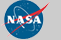Curator: David M. Giles NASA Official: Brent N. Holben Generated: 13/12/2019